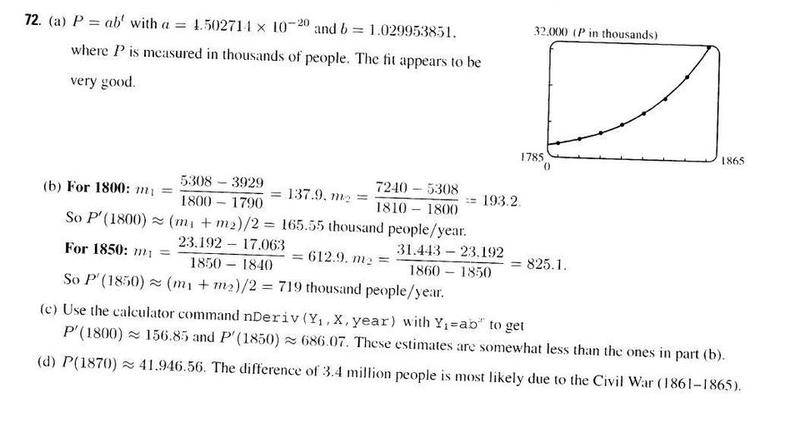# Derivative Word Problem - US Population

## Homework Statement

(a) Use a graphing calculator or computer to fit an exponential function to the data. Graph the data points and the exponential model. How good is the fit?

(b) Estimate the rates of population growth in 1800 and 1850 by averaging slopes of secant lines.

(c) Use the exponential model in part (a) to estimate the rates of growth in 1800 and 1850. Compare these estimates with the ones in part (b).

(d) Use the exponential model to predict the population in 1870. Compare with the actual population of 38,558,000. Can you explain the discrepancy?

Year Population
1790 3,929,000
1800 5,308,000
1810 7,240,000
1820 9,639,000
1830 12,861,000
1840 17,063,000
1850 23,192,000
1860 31,443,000

## The Attempt at a Solution

I understand part B, you would just do the population in 1800 - population in 1790 all divded by 1800 - 1790... etc. etc..

However, part A and consequently parts C and D are confusing me...

Any help would be GREATLY appreciated.

Thanks,
Bob

Dick
Homework Helper
You are supposed to use a computer or calculator to do a best fit exponential to the data. You could do this by hand, but it would be tedious and they don't want that. Once you have that fit then you have a model of uniform growth rate over that time. The actual growth rate over any single decade may differ from that average. In particular for d) you are going to find the population growth rate is considerably smaller than you might expect for the period 1860-1870. Did something happen in the US in the decade that might explain it? It's all just about comparing an average growth model with individual data points and figuring out why some of them might not fit the average behavior.

Ok, but how exactly would I fix an exponential to the data?

Our teacher gave us the solution as this:Perhaps you could explain exactly what they did?

How did they get those numbers in part a?

Dick
Homework Helper
They typed the population numbers into a calculator or a computer program. They expect you to be able to do the same. Don't you have one or the other?

Last edited:
Yeah, ha ha, but excuse my "noobness". This is my first calculus course and am still trying to figure out my TI-89.

How would I graph the population numbers on my calculator? Would I use the table feature?

Bob

Dick
Homework Helper
Yeah, ha ha, but excuse my "noobness". This is my first calculus course and am still trying to figure out my TI-89.

How would I graph the population numbers on my calculator? Would I use the table feature?

Bob

Sorry, I can't help you there. I don't own a calculator that does much more than multiply or divide. I did google and find this. http://www.prenhall.com/esm/app/cal...cuments/TI-89/desc_pages/ti89techskills2.html Does that help? If not I'm sure you can find lots of other stuff on the web.

Last edited:
Sorry, I can't help you there. I don't own a calculator that does much more than multiply or divide. I did google and find this. http://www.prenhall.com/esm/app/cal...cuments/TI-89/desc_pages/ti89techskills2.html Does that help? If not I'm sure you can find lots of other stuff on the web.

I tried following the instructions on site to plot the points on my TI89 but to no avail.

Any further help is kindly welcome.

-Bob

Dick
Homework Helper
I'll post a call for help from other homework helpers who might know this stuff.

D H
Staff Emeritus
What instructions did you follow? Those for a linear regression? You are supposed to do an exponential fit. The section of the web page Dick found on nonlinear regression talks about exactly this kind of problem.

What instructions did you follow? Those for a linear regression? You are supposed to do an exponential fit. The section of the web page Dick found on nonlinear regression talks about exactly this kind of problem.

Thank you! I've finally figured it out thanks to the instructions that Dick linked to on that site. Thank you so much for pointing that out.

Ha, I am super happy right now you all have no idea. I was trying to figure this out forever, thank you!

-Bob

Redbelly98
Staff Emeritus